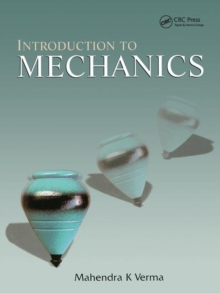Supporting your high street Find out how »
• My AccountIntroduction to Mechanics Paperback / softback

Description

A modern introduction to Newtonian dynamics and the basics of special relativity, this book discusses standard topics such as Newton's laws of motion, energy, linear and angular momentum, rigid body dynamics, and oscillations, then goes on to introduce modern topics such as symmetries, phase space, nonlinear dynamics and chaos.

The author presents Newton's equation of motion as a differential equation, bringing out key issues such as phase space and determinism in mechanical systems and helps introduce modern research topics such as chaos theory in a natural way.

He highlights key assumptions of Newtonian mechanics and incorporates numerical solutions of many mechanical systems using MATLAB (R).

Information

• Format: Paperback / softback
• Pages: 356 pages
• Publisher: Taylor & Francis Ltd
• Publication Date:
• Category: Applied physics
• ISBN: 9781138116771

Other Formats

£59.99

£53.19

on all orders

Pick up orders

from local bookshops

£165.00

£151.35